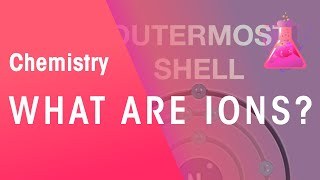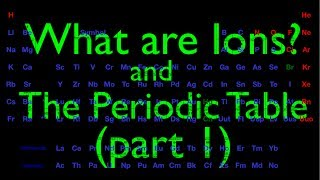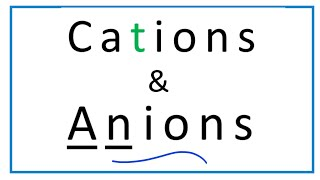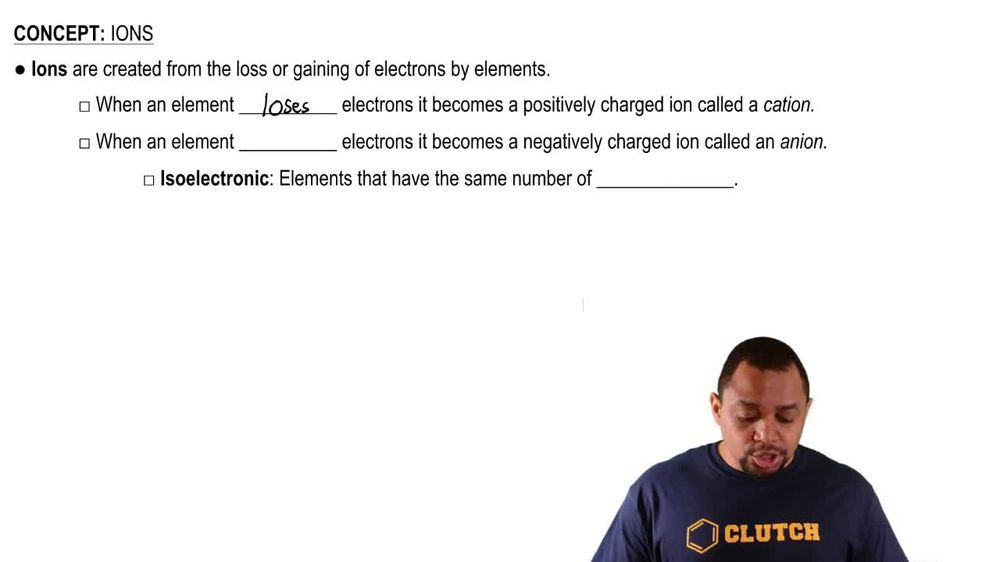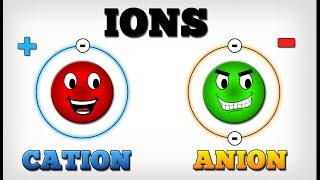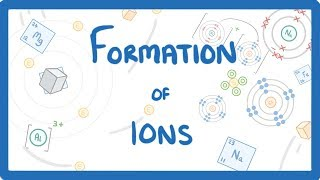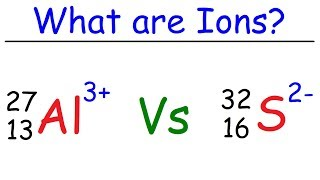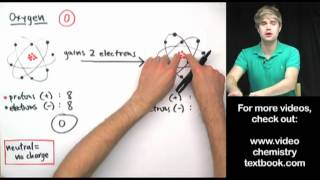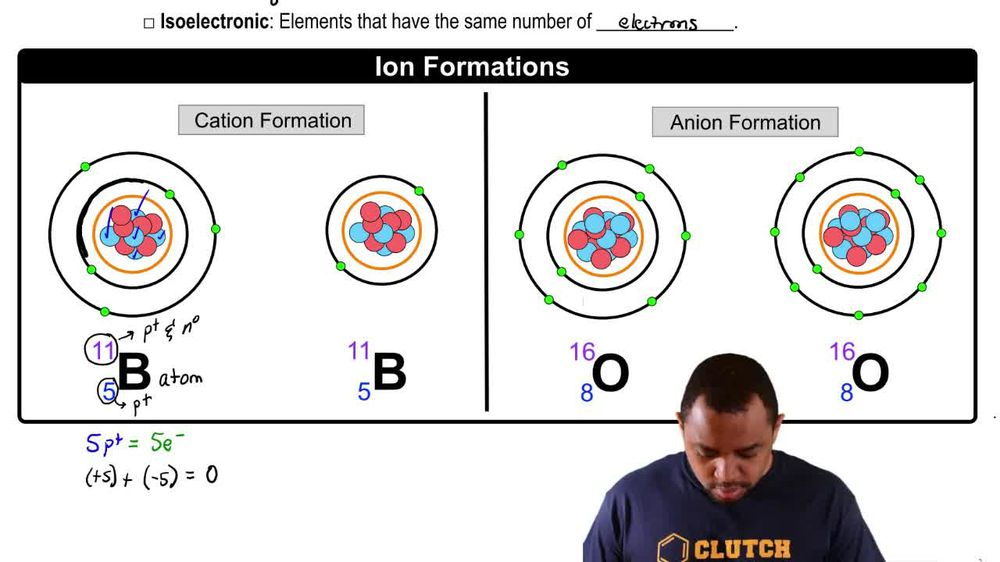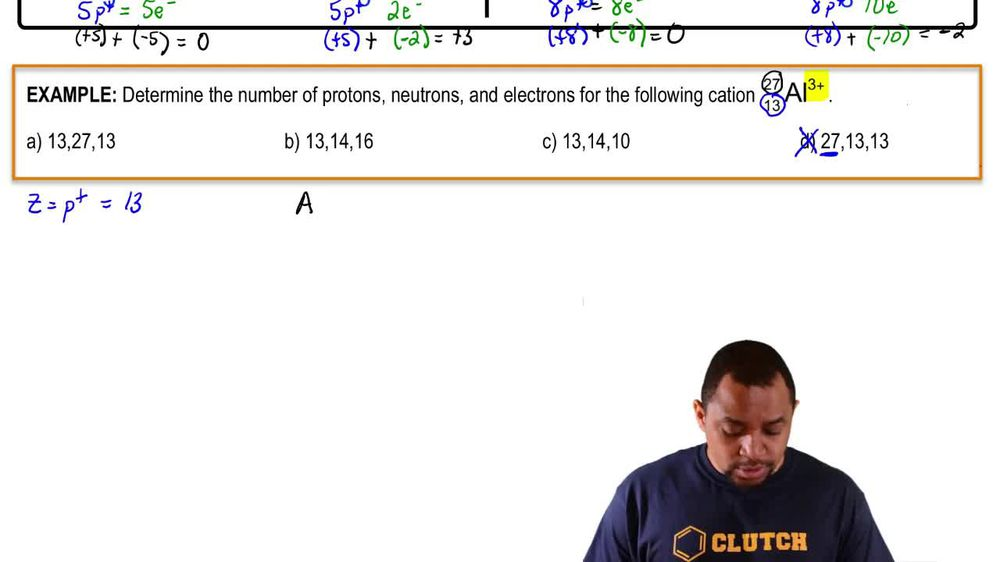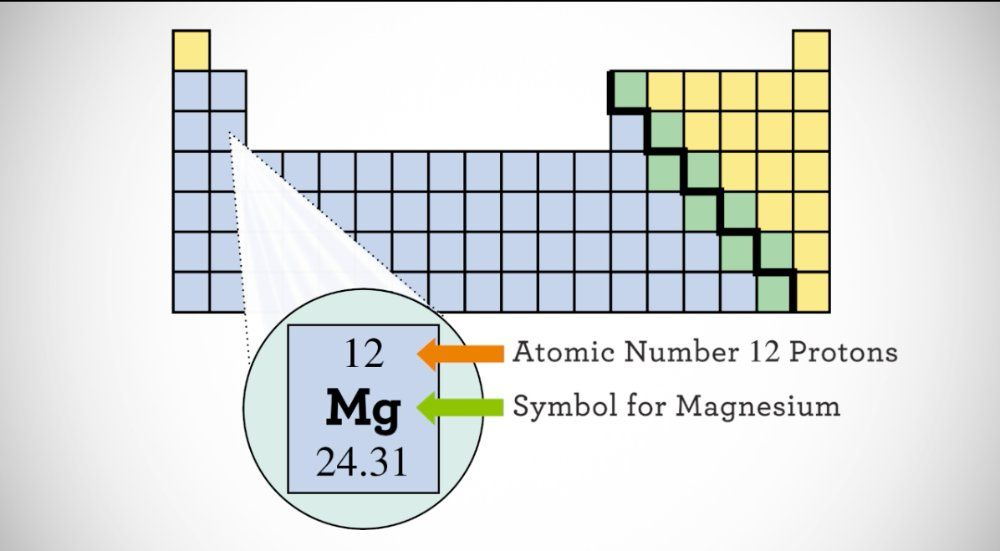Start typing, then use the up and down arrows to select an option from the list.
1. 2. Atoms & Elements2. Ions
Problem

# How many protons and electrons are in each of the following ions? (a) Be2+ b) Rb+ c) Se2- (d) Au3+

Relevant Solution2m
Play a video:
hey everyone in this example, we need to count the number of electrons and protons in our given ion. In this case C. 03 plus we want to go ahead and recall a few facts. The first being that our atomic number which can be represented by the term Z, is equal to our number of protons in any atom. The next thing we want to recall is that for neutral atoms only our number of protons are equal to our number of electrons. However, if we don't have a neutral atom, so for charged atoms or ions in this case we would say that our number of electrons are equal to our atomic number minus our charge. And so let's go ahead and get into how many protons we have in cobalt. So we're going to find cobalt on our periodic tables and we see that it's going to be in Group eight B of the transition metals. So we should recognize that this also comes with the atomic number In our information for cobalt. And we see that Z or atomic number is equal to 27. And so therefore we can say we have 27 protons and cobalt Or in our cobalt three plus Catalon. Our next step is to count our electrons in cobalt. So a number of electrons in cobalt. And so this is going to be equal to our number of protons Z. Which we just found to be 27 and taking the difference of our electron charge. So our electron charge is a plus three. We know that a positive charge means that we will lose those amount of electrons and so we would lose three electrons. So it makes sense that we do 27 minus our charge of plus three. So we have 27 -3. And this difference gives us a total of 24 electrons. And so this completes this example As our number of protons and electrons for our cobalt three plus cast iron. So I hope that everything we reviewed was clear. But if you have any questions, leave them down below and I will see everyone in the next practice video.